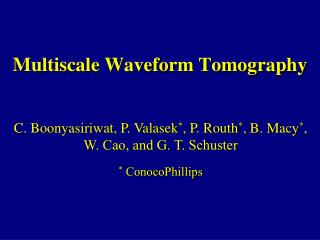DownloadDownload PresentationMultiscale Waveform Tomography

# Multiscale Waveform Tomography

Télécharger la présentation## Multiscale Waveform Tomography

- - - - - - - - - - - - - - - - - - - - - - - - - - - E N D - - - - - - - - - - - - - - - - - - - - - - - - - - -
##### Presentation Transcript

1. Multiscale Waveform Tomography C. Boonyasiriwat, P. Valasek*, P. Routh*, B. Macy*, W. Cao, and G. T. Schuster * ConocoPhillips

2. Outline • Goal • Introduction • Theory of Acoustic Waveform Tomography • Multiscale Waveform Tomography • Results • Conclusions 1

3. Goal 2

4. Outline • Goal and Motivation • Introduction • Theory of Acoustic Waveform Tomography • Multiscale Waveform Tomography • Results • Conclusions 3

5. Introduction ? 4

6. Introduction: Waveform Tomography • No high frequency approximation • Frequency domain: Pratt et al. (1998), etc. • Time domain: Zhou et al. (1995), Sheng et al. (2006), etc. • Pratt and Brenders (2004) and Sheng (2006) used early-arrival wavefields. • Bunks et al. (1995) and Pratt et al. (1998) used multiscale approaches. 10

7. Outline • Goal • Introduction • Theory of Acoustic Waveform Tomography • Multiscale Waveform Tomography • Results • Conclusions 11

8. Why Acoustic? • Elastic wave equation is expensive. • Waveform inversion is also expensive. • Previous research shows acoustics is adequate. • Use acoustics and mute unpredicted wavefields 12

9. Theory of Waveform Tomography The waveform misfit function is An acoustic wave equation: 13

10. Theory of Waveform Tomography The steepest descend method is used to minimize the misfit function: The waveform residual is defined by 14

11. Theory of Waveform Tomography where The gradient is calculated by 15

12. Outline • Goal • Introduction • Theory of Acoustic Waveform Tomography • Multiscale Waveform Tomography • Results • Conclusions 16

13. Why using Multiscale? Misfit function ( f ) Model parameter (m) Low Frequency Coarse Scale High Frequency Fine Scale Image from Bunk et al. (1995) 17

14. Our Multiscale Approach • Combine Early-arrival Waveform Tomography (Sheng et al., 2006) and a time-domain multiscale approach (Bunk et al., 1995) • Use a Wiener filter for low-pass filtering. • Use an early-arrival window function to mute all energy except early arrivals. • Use multiscale V-cycles. 18

15. Multiscale V-Cycle High Frequency Fine Grid Low Frequency Coarse Grid 19

16. Why a Wiener Filter? Target Wavelet Original Wavelet Wavelet: Hamming Window Wavelet: Wiener Filter 20

17. Outline • Goal • Introduction • Theory of Acoustic Waveform Tomography • Multiscale Waveform Tomography • Results • Conclusions 21

18. Synthetic SSP Data Results • Three-Layer Model • Layered Model with Scatters • SEG Salt Model • Zhu’s Model • Mapleton Model 22

21. MWT Tomogram (5,10 Hz) Gradient 27

23. EWT Tomogram using 15-Hz Data Gradient 32

24. MWT Tomogram using 2.5-Hz Data Gradient 33

25. MWT Tomogram using 5-Hz Data 2.5-Hz 34

26. MWT Tomogram using 15-Hz Data 10 Hz 36

27. Comparison of Misfit Function 15 Hz 15 Hz 5 Hz 10 Hz 2.5 Hz 38